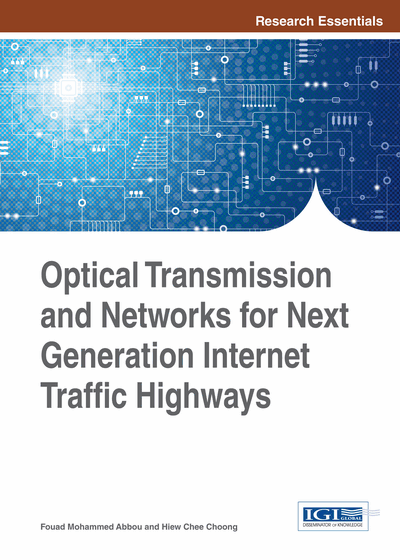# Network Performance Analysis with Nonlinear Effects

DOI: 10.4018/978-1-4666-6575-0.ch015
Available
\$37.50
No Current Special Offers

## Abstract

The nonlinear Bit Error Rate (BER) performance of dense Wavelength Division Multiplexed (WDM) Manhattan Street Networks with deflection routing was obtained using a semi-analytical model. The chapter's results show that nonlinear effects impose significant performance penalties on dense WDM networks, both in terms of maximum hops attainable and average BER, and should be taken into account when modeling such networks. Simple techniques such as optimal amplifier positioning can mitigate some of the nonlinear penalties.
Chapter Preview
Top

## System Model

The Hot-Potato MS network with deflection routing is described using the deflection probability p(g) given the packet generation probability or network load g (Forghieri, Bononi, & Prucnal, 1995). Using p(g), the hop distribution P(n), which is the probability density function of the number of hops, n required to travel to the destination can be obtained. The process of obtaining P(n) and p for given values of g is elaborated in (Forghieri, Bononi, & Prucnal, 1995), and is not repeated here. In addition, the BER of the packets depends on the node (cross-connect) structure and the fiber link between nodes. The node structure used is shown in Figure 1(a).

All transmitters are assumed to transmit with the same average power, PTX, which is the signal power of a WDM channel. Each signal consists of a pseudorandom bit sequence (PRBS) of 16384 bits where the modulation format is RZ-OOK (return-to-zero-on-off-keying) with 50% pulse duty cycle and a bit rate BR of 42.656 GHz. We consider a high capacity, dense WDM network where the spectral density (spectral efficiency) is high at 0.4, corresponding to a channel spacing of 107 GHz. The WDM demultiplexers are modeled as second order Gaussian filters centered at the WDM channels’ center frequencies. The total node loss is

(15.1) (Castanon, Tonguz, & Bononi, 1997). The receivers are direct-detection receivers followed by a fifth order Bessel postdetection electrical filter. The main switch introduces crosstalk noise, because a portion of an output link signal leaks onto the other output link, and is specified by the leakage parameter, ε. This causes in-band crosstalk, which is modeled as additive colored Gaussian noise over an effective noise bandwidth BN, with noise power given by (Castanon, Tonguz, & Bononi, 1997).if i =1otherwise (15.2) where i is the current node, Pin,switch is the power at the switch inputs, u is the link utilization and a is the absorption rate defined in (Forghieri, Bononi, & Prucnal, 1995).

## Complete Chapter List

Search this Book:
Reset